#### 期刊菜单

Analysis of Influence of Distributed Generation Supply Access on Current Protection of Distribution Network
DOI: 10.12677/SG.2017.75044, PDF, HTML, XML, 下载: 1,363  浏览: 2,817

Abstract: A large number of distributed generation (DG) access will change the structure of the traditional distribution network and affect the current protection of distribution network. Based on the analysis of the current protection system in traditional distribution network, this paper analyzes the influence of the DG penetration on the current protection system of distribution network. Focusing on the problems include current protection sensitivity reduction or protection system malfunction and refusal with DG penetration, this paper proposed a solution for current protec-tion configuration and parameter tuning for distribution network penetrated with DG. Also, taking the distribution network with DG in Shenzhen 10 kV regional as an example, the paper analyzes the malfunction of the protection system caused by DG penetration. Then, the effectiveness of the proposed solution is verified and results reveal that the proposed solution can provide meaningful reference for distribution network protection system penetrated with DG.

1. 引言

2. 配电网中电流保护配置情况

1) 电流速断保护(电流 I 段保护)

${I}_{{k}_{1}}^{\text{I}}={K}_{rel}^{\text{I}}{I}_{B\mathrm{max}}$ (1)

${K}_{rel}^{Ι}$ ：电流速断保护可靠系数，一般取1.2~1.3；

${I}_{B\mathrm{max}}$ ：B点短路时的最大短路电流，一般为三相短路电流(A)。

$\frac{{l}_{\mathrm{min}}}{{l}_{AB}}=\frac{1}{{Z}_{AB}}\left(\frac{\sqrt{3}}{2}\frac{{E}_{\phi }}{{I}_{{k}_{1}}^{\text{I}}}-{z}_{s\mathrm{max}}\right)$ (2)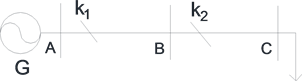Figure 1. Schematic of the Protection k1

${l}_{AB}$ ：线路AB长度(m)；

${z}_{AB}$ ：线路AB的阻抗(Ω)；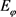：系统等效电源相电动势(V)；

${z}_{s\mathrm{max}}$ ：系统最小运行方式下阻抗(Ω)。

2) 限时电流速断保护(电流 II 段保护)

${I}_{{k}_{1}}^{\text{II}}={K}_{rel}^{\text{II}}\cdot {I}_{{k}_{2}}^{\text{I}}$ (3)

${K}_{rel}^{\text{II}}$ ：限时电流速断保护的可靠系数，一般取1.1~1.2；

${I}_{{k}_{2}}^{I}$ ：保护k2的电流速断保护整定值(A)。

${t}_{{k}_{1}}^{\text{II}}={t}_{{k}_{2}}^{I}+\Delta t$ (4)

${K}_{sen{k}_{1}}=\frac{{I}_{B\mathrm{min}}}{{I}_{{k}_{1}}^{\text{II}}}$ (5)

${I}_{B\mathrm{min}}$ ：系统最小运行方式下，线路AB末端发生两相短路时，流过k1的短路电流(A)；

3) 定时限过电流保护(电流 III 段保护)

${I}_{{k}_{1}}^{\text{III}}=\frac{{K}_{rel}^{\text{III}}{K}_{ss}}{{K}_{re}}{I}_{AB\mathrm{max}}$ (6)

${K}_{ss}$ ：自启动系数，由网络构架和负荷性质决定；

${K}_{re}$ ：继电器返回系数，一般取0.85~0.95；

${I}_{AB\mathrm{max}}$ ：线路AB正常运行时的最大负荷电流(A)。

${t}_{{k}_{1}}^{\text{III}}={t}_{{k}_{2}}^{\text{III}}+\Delta t$ (7)

${K}_{sen{k}_{1}}^{\text{III}}=\frac{{I}_{\mathrm{min}}}{{I}_{{k}_{1}}^{\text{III}}}$ (8)

${I}_{{k}_{1}}^{\text{III}}$ ：保护k1定时限过电流保护整定值(A)；

${I}_{\mathrm{min}}$ ：近后备时为最小运行方式下，线路AB末端两相短路时的电流(A)；远后备时为最小运行方式下，线路BC末端两相短路的电流(A)。

3. 分布式电源接入对三段式电流保护的影响及解决方案

1) 增大保护系统灵敏度，使保护失去选择性。当分布式电源接入的下游线路或相邻线路发生短路故障时，分布式电源将与大电网共同提供短路电流，使得某些保护装置检测的短路电流增大，将增大其保护灵敏度，严重时保护范围将延伸到下一线路，使保护失去选择性。

2) 降低保护系统灵敏度。由于分布式电源的分流作用，可能使得分布式电源所在线路保护装置检测的短路电流值减小，降低其保护灵敏度，严重时，保护装置可能发生拒动。

3) 引起保护系统误动作。在相邻线路发生短路时，分布式电源所在线路的保护装置将检测到由分布式电源提供的反向短路电流，可能使得保护装置不分方向误动作而切除。

4. 案例分析

4.1. 增大保护灵敏度，使保护失去选择性

${K}_{rel}^{Ι}=1.2$${E}_{\Phi }=1$${z}_{s}=0.1$${z}_{AD}={z}_{DE}=1$ 。由前述(1)可得，未接入DG前，k4电流速断保护整定值为：

${I}_{{k}_{4}}^{Ι}={K}_{rel}^{Ι}\frac{{E}_{\Phi }}{{z}_{s}+{z}_{AD}+{z}_{DE}}=1.2×\frac{1}{0.1+1+1}=0.57$

${{I}^{\prime }}_{{k}_{4}}=\frac{1}{\frac{\left({z}_{s}+0.8{z}_{AD}\right)×{z}_{DG}}{{z}_{s}+0.8{z}_{AD}+{z}_{DG}}+0.2{z}_{AD}+{z}_{DE}}=\frac{0.9+{z}_{DG}}{1.08+2.1{z}_{DG}}$ (9)Figure 2. 10 kV distribution network with the distributed generation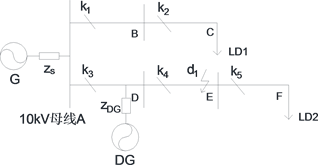Figure 3. Three-phase short circuit happen in d1 of the end of line DE

${z}_{DG}=0.13×\frac{{S}_{B}}{{S}_{DG}}=\frac{13}{{S}_{DG}}$ (10)

${{I}^{\prime }}_{{k}_{4}}=\frac{13+0.9{S}_{DG}}{27.3+1.08{S}_{DG}}$ (11)

4.2. 降低保护灵敏度

${{I}^{″}}_{{k}_{4}}=\frac{\sqrt{3}}{2}\frac{1}{\frac{\left({z}_{s}+0.8{z}_{AD}\right)×{z}_{DG}}{{z}_{s}+0.8{z}_{AD}+{z}_{DG}}+0.2{z}_{AD}+{z}_{DE}}=\frac{\sqrt{3}}{2}\frac{0.9+{z}_{DG}}{1.08+2.1{z}_{DG}}$ (12)

${{I}^{\prime }}_{{k}_{3}}=\frac{{z}_{DG}}{{z}_{s}+{z}_{DG}+0.8{z}_{AD}}×{{I}^{″}}_{{k}_{4}}=\frac{\sqrt{3}{z}_{DG}}{2.16+4.2{z}_{DG}}$ (13)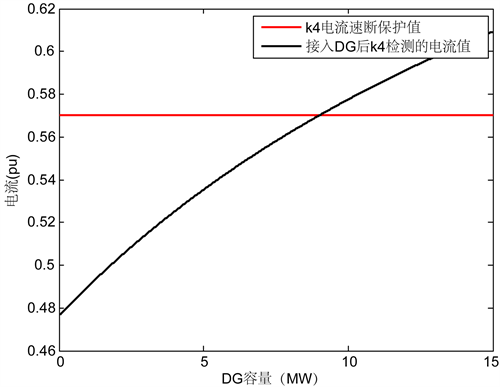Figure 4. The short circuit current detected by the protective device k4 varies with the DG capacity variation curve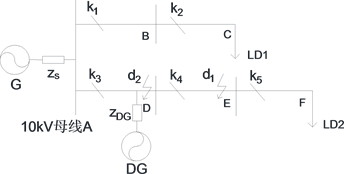Figure 5. Two-phase short circuit happen in d1 of the end of line DE(14)

4.3. 引起保护误动作Figure 6. The short circuit current detected by the protective device k3 varies with the DG capacity variation curveFigure 7. Three-phase short circuit happen in d3 of the end of line AB

${z}_{AB}=1$ ，则可知k1检测的短路电流为：

${{I}^{\prime }}_{{k}_{1}}=\frac{1}{\frac{\left(0.8{z}_{AD}+{z}_{DG}\right)×{z}_{s}}{0.8{z}_{AD}+{z}_{DG}+{z}_{s}}+{z}_{AB}}=\frac{0.9+{z}_{DG}}{0.98+1.1{z}_{DG}}$ (15)

${{I}^{″}}_{{k}_{3}}=\frac{{z}_{s}}{{z}_{s}+0.8{z}_{AD}+{z}_{DG}}×{{I}^{\prime }}_{{k}_{1}}=\frac{0.1}{0.98+1.1{z}_{DG}}$ (16)

${{I}^{″}}_{{k}_{3}}=\frac{0.1{S}_{DG}}{14.3+0.98{S}_{DG}}$ (17)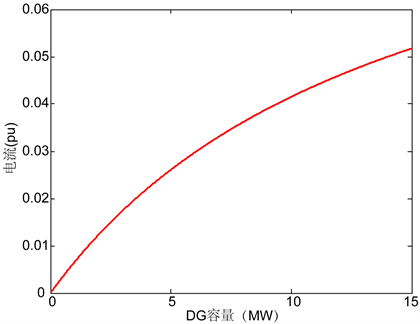Figure 8. The protection device k3 detects the reverse short-circuit current with the DG capacity change curve

4.4. 小结

5. 分布式电源接入对自动重合闸的影响

6. 结论

 王敏, 丁明. 含分布式电源的配电系统规划[J]. 电力系统及其自动化学报, 2004, 16(6): 5-8.

 陈玉伟, 谢荣, 于泳. 分布式电源接入配电网容量及保护研究[J]. 电工电气, 2014(1): 20-23.

 夏熠. 分布式电源接入对配电网的影响及保护方法探究[D]: [硕士学位论文]. 合肥: 合肥工业大学, 2015.

 周卫, 张尧, 夏成军, 等. 分布式发电对配电网继电保护的影响[J]. 电力系统保护与控制, 2010, 38(3): 1-5.

 卫波. 分布式电源接入对配电网继电保护的影响及对策的研究[D]: [硕士学位论文]. 北京: 华北电力大学, 2013.

 洪虹, 杨升峰, 朱宁辉. 分布式电源接入对配电网继电保护的影响[J]. 电气时代, 2013(11): 70-71.

 马超. 分布式电源接入对配电网继电保护的影响研究[D]: [硕士学位论文]. 新疆: 新疆农业大学, 2015.

 温阳东, 王欣. 分布式发电对配电网继电保护的影响[J]. 电力系统保护与控制, 2008, 36(1): 12-14.

 尹项根, 张哲, 肖繁, 等. 分布式电源短路计算模型及电网故障计算方法研究[J]. 电力系统保护与控制, 2015, 43(22): 1-9.

 王希舟, 陈鑫, 罗龙, 等. 分布式发电与配电网保护协调性研究[J]. 电力系统保护与控制, 2006, 34(3): 15-19.

 Kumpulainen, L.K. and Kauhaniemi, K.T. (2004) Analysis of the Impact of Distributed Generation on Automatic Reclosing. Power Systems Conference & Exposition, 603-608. https://doi.org/10.1109/PSCE.2004.1397623

 刘魁元. 分布式电源对配电网自动重合闸的影响研究[D]: [硕士学位论文]. 北京: 北京交通大学, 2012.

  王敏, 丁明. 含分布式电源的配电系统规划[J]. 电力系统及其自动化学报, 2004, 16(6): 5-8.  陈玉伟, 谢荣, 于泳. 分布式电源接入配电网容量及保护研究[J]. 电工电气, 2014(1): 20-23.  夏熠. 分布式电源接入对配电网的影响及保护方法探究[D]: [硕士学位论文]. 合肥: 合肥工业大学, 2015.  周卫, 张尧, 夏成军, 等. 分布式发电对配电网继电保护的影响[J]. 电力系统保护与控制, 2010, 38(3): 1-5.  卫波. 分布式电源接入对配电网继电保护的影响及对策的研究[D]: [硕士学位论文]. 北京: 华北电力大学, 2013.  洪虹, 杨升峰, 朱宁辉. 分布式电源接入对配电网继电保护的影响[J]. 电气时代, 2013(11): 70-71.  马超. 分布式电源接入对配电网继电保护的影响研究[D]: [硕士学位论文]. 新疆: 新疆农业大学, 2015.  温阳东, 王欣. 分布式发电对配电网继电保护的影响[J]. 电力系统保护与控制, 2008, 36(1): 12-14.  尹项根, 张哲, 肖繁, 等. 分布式电源短路计算模型及电网故障计算方法研究[J]. 电力系统保护与控制, 2015, 43(22): 1-9.  王希舟, 陈鑫, 罗龙, 等. 分布式发电与配电网保护协调性研究[J]. 电力系统保护与控制, 2006, 34(3): 15-19.  Kumpulainen, L.K. and Kauhaniemi, K.T. (2004) Analysis of the Impact of Distributed Generation on Automatic Reclosing. Power Systems Conference & Exposition, 603-608. https://doi.org/10.1109/PSCE.2004.1397623  刘魁元. 分布式电源对配电网自动重合闸的影响研究[D]: [硕士学位论文]. 北京: 北京交通大学, 2012.